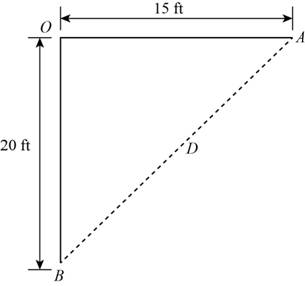# The function that models the distance ( D ) between the ships in terms of time t ( in hours ) elapsed since their departure.### Precalculus: Mathematics for Calcu...

6th Edition
Stewart + 5 others
Publisher: Cengage Learning
ISBN: 9780840068071### Precalculus: Mathematics for Calcu...

6th Edition
Stewart + 5 others
Publisher: Cengage Learning
ISBN: 9780840068071

#### Solutions

Chapter 2, Problem 13P
To determine

## To find: The function that models the distance (D) between the ships in terms of time t(in hours) elapsed since their departure.

Expert Solution

The function that models distance between ships in terms of time (in hours) is D(t)=25t,t>0 .

### Explanation of Solution

Given:

Speed of ship sailing south is 15mi/h

Speed of ship sailing east is 20mi/h

Calculation:

Draw the Figure (1) as shown below after time t since departure of ships.Figure (1)

In Figure (1) O is starting point of ships, A,B are the points where ship sailing towards south and east reach after time t respectively.

Calculate distance OA and OB shown in Figure (1) as,

OA=15t[Distance=Speedtime]

And,

OB=20t[Distance=Speedtime]

Apply Pythagorean theorem in triangle OAB shown in Figure (1)

(AB)2=(OA)2+(OB)2

Substitute D for AB , 15t for OA and 20t for OB in above equation.

(D)2=(15t)2+(20t)2D2=225t2+400t2

Factor (t2) from right hand side of the above equation.

D2=t2(225+400)=t2(625)

Take square root of above equation.

D=25t

Thus, function that models distance between ships in terms of time (in hours) is D(t)=25t,t>0 .

### Have a homework question?

Subscribe to bartleby learn! Ask subject matter experts 30 homework questions each month. Plus, you’ll have access to millions of step-by-step textbook answers!